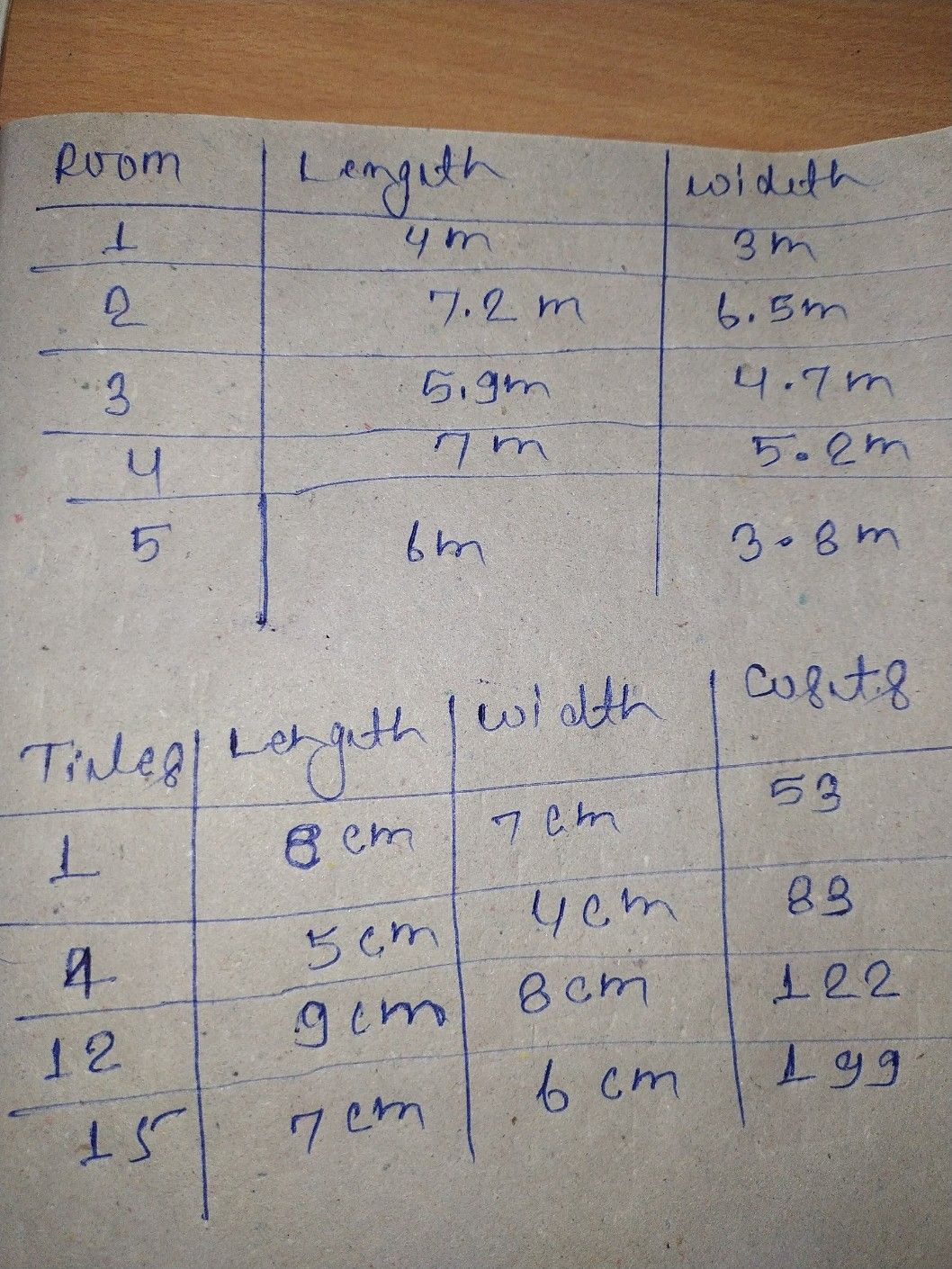Symbol
ProblemActivity $3$ $-$ $D1rections$ Perform the following activity. $1$ Find the dimensions of the floors of at least two rooms in your school. Indicate the measures obtained $in$ the table $below$ Rooms Length Width $2$ $D$ Determine the measures and $costs$ of different tiles that are available in the nearest hardware store or advertised in any printed materials or in the internet. Write these in the table below. $T1les$ Length Width $Costs$
7th-9th grade
Other
Search count: 105
SolutionQanda teacher - ShubhamSonStudent
thank you ma'am❤